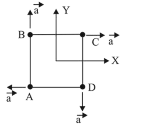# Four particles A, B, C and D with massesQuestion:

Four particles A, B, C and D with masses $m_{\text {A }}=\mathrm{m}, m_{\text {B }}=2 \mathrm{~m}$, $m_{\mathrm{C}}=3 \mathrm{~m}$ and $m_{\mathrm{D}}=4 \mathrm{~m}$ are at the corners of a square. They have accelerations of equal magnitude with directions as shown. The acceleration of the centre of mass of the particles is:1. (1) $\frac{a}{5}(\hat{i}-\hat{j})$

2. (2) $a(\hat{i}+\hat{j})$

3. (3) Zero

4. (4) $\frac{a}{5}(\hat{i}+\hat{j})$

Correct Option: 1

Solution:

(1) Acceleration of centre of mass $\left(a_{\mathrm{cm}}\right)$ is given by

$\therefore \vec{a}_{\mathrm{cm}}=\frac{m_{1} \vec{a}_{1}+m_{2} \vec{a}_{2}+\ldots \ldots \ldots}{m_{1}+m_{2}+\ldots \ldots . .}$

$=\frac{(2 m) a \hat{j}+3 m \times a \hat{i}+m a(-\hat{i})+4 m \times a(-\hat{j})}{2 m+3 m+4 m+m}$

$=\frac{2 a \hat{i}-2 a \hat{j}}{10}=\frac{a}{5}(\hat{i}-\hat{j})$